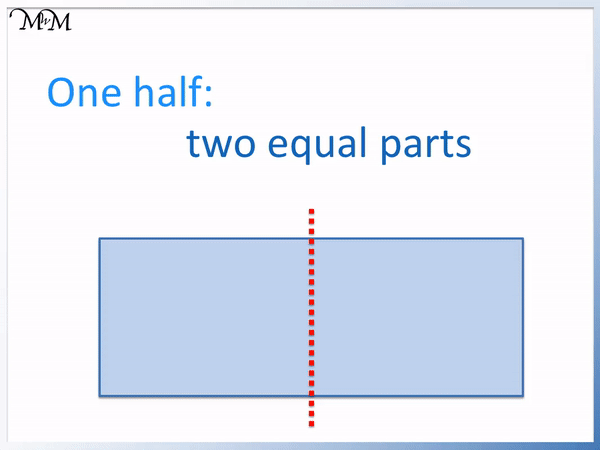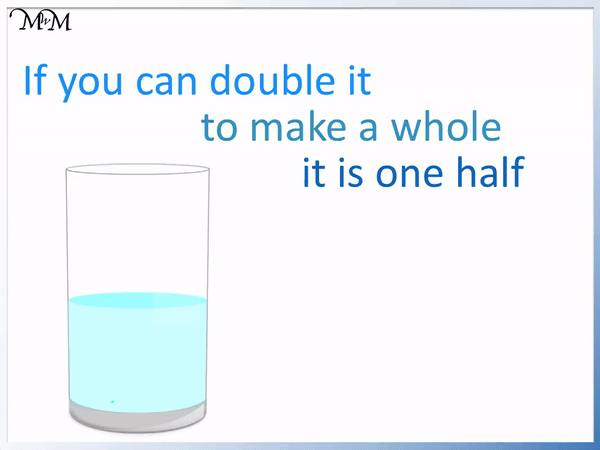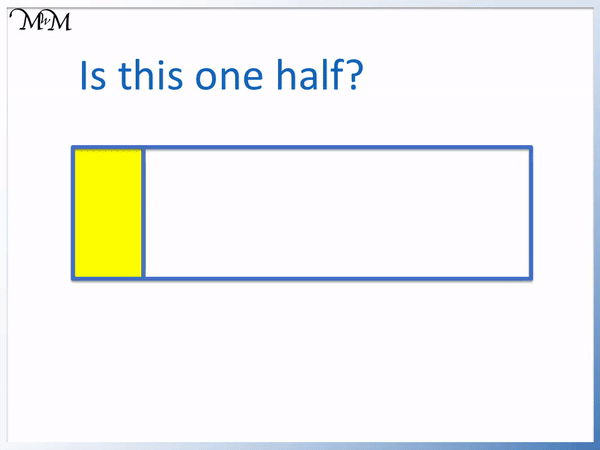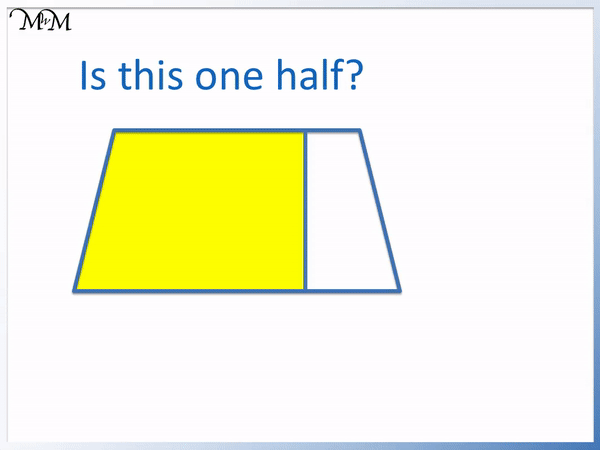# Finding Half of a Shape

Finding Half of a Shape• To find half of a shape divide it exactly into two equal parts.
• The line in the example above divides the shape into two parts which are both exactly the same size.
• To show one half, we colour one side of this dividing line.
• We have a half if two of these parts fit into the whole shape.
• We have coloured in 1 out of 2 equal parts.
• One half is written as a fraction by writing 1 out of 2 as   1 / 2  .

A half is one of two equally-sized parts.

We have half of a shape if we can fit two of these parts into the whole shape exactly.• We are asked to decide if the shaded section of the shape is one half or not.
• If a shaded section is one half then it will fit exactly twice into the whole shape.
• If we add another of these sections, the whole shape is still not complete.
• The shaded section is less than one half.
• If the shaded section was one half then the whole shape would be divided into two equally sized sections.
• We can see that the shaded section is smaller than the other unshaded section and that they are not equal in size.Supporting Lessons# Finding Half of a Shape

In this lesson we learn how to recognise one half of a shape and how to decide if a shaded section is half of a shape or not.

One half is one of two equally sized parts.

Below is an example of splitting a rectangle into two equal halves.The rectangle above is split into two halves by dividing it down the middle.

The rectangle is divided so that there are a total of 2 parts and both parts are exactly the same size.When teaching finding and recognising one half of a shape, the most common mistake is that a child will misidentify a half because they did not check that both parts are of an equal size.

In order to decide if we have half of a shape, we must check if there are two sections and if they are both the same size.

Just because the shape is split into two sections does not mean that we have a half.

Below is another rectangle.We can divide it through the middle, into two equal parts.

The top part is shaded in and it is exactly equal in size to the part on the other side of the dividing line. Therefore the shaded section is one half.

We have one out of two equal parts.

We can write one half as a fraction   1 / 2.

This fraction can be read as ‘one out of two’. We can recognise this fraction as being called one half.

Below is a triangle and we will shade in one half of it.

To shade in a half we need to divide it into two equal sections.

A common technique in primary school questions is to try and split the shape vertically or horizontally down the middle.The darker coloured side on the left is exactly the same size as the yellow shaded triangle on the right.

When teaching shading one half, it can be useful to first look for lines of symmetry and you can get a mirror or fold the shape along the dividing line. If it looks the same, we know it is a line of symmetry.

If we have a line of symmetry, we have one half. However, just because we have a half does not always mean that the dividing line is a line of symmetry.

We are sometimes asked to shade in or draw one half of a real life object.

Below we have a half full glass of water.We know that we have a half if it fits exactly into the whole shape twice.

Another way to teach this is that we can double our half to make a whole.

If we double the amount of water in the glass, it fills the whole glass.

In the example below, we are asked to decide if the shaded section is equal to one half of the rectangle shape.

If we double the shaded shape, we can see that it does not fit the whole rectangle.We can double the shaded section and it is still less than the whole rectangle shape.

And so, the shaded section is less than one half.

The easiest check to do is to look at the two original parts that the whole rectangle has been divided into and to decide if they are equal in size.

The shaded section is smaller than the section of the rectangle that is not shaded and so, the shaded section is less than one half.

Here is another example of deciding if we have one half of a shape or not.

We can double the original shaded section to see.Two of the shaded sections are more than the whole shape.

The shaded section is more than one half.

We can see that the original shaded section is larger than the section that is unshaded. This shape is not divided into two equal partsNow try our lesson on Introduction to Halving Numbers where we learn how to halve numbers.